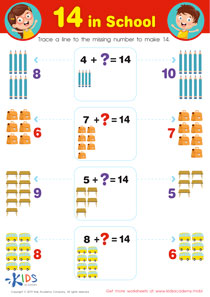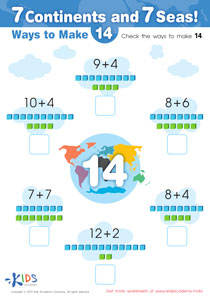# Math Lesson - Making 14, Kindergarten

• ### Activity 1 / 14 in School WorksheetBefore beginning this worksheet, you can first remind your kids that adding different sets of numbers can give you the same total. Give them simple examples, like 2+3 =5, and also 4+1 =5. Now, test your children’s understanding by asking them to give you some more examples. Then, you can work through this exercise. Help them count the objects, and then trace a line to the missing number that makes the total 14.

• ### Activity 2 / 7 Continents and 7 Seas WorksheetBefore starting this worksheet, remind your kids the different continents we have in the world. Now, teach them that different numbers added together can give the same figure. Give your kids simple equations, like adding 2+2 =4, and also 3+1 =4. Ask your students if they can give you other examples, and then look at the simple additions in this printout. Help your students work them all out, and then circle all of the equations that make 14.

?